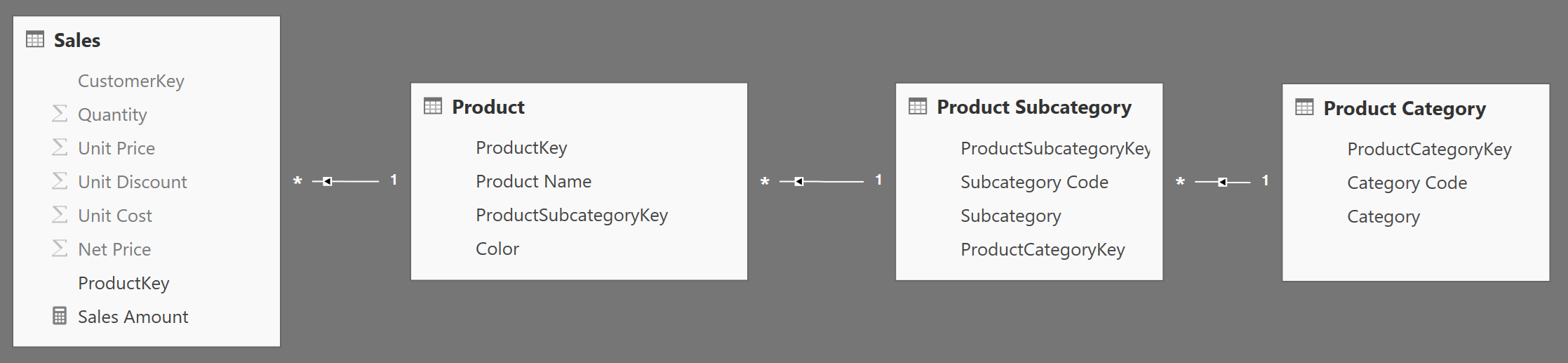In DAX, columns have lineage. Knowing when lineage is maintained in a DAX expression and when it is not, is an important skill to write effective code. As a general rule, column references maintain lineage whereas expressions lose it. This puzzle is a single and simple question, but you have to find the exact answer.

Scenario
The data model is straightforward: you have Sales and the usual chain of relationship through Product, Subcategory and Category.## The Challenge

You know the result of the following calculated table:

```EVALUATE
SUMMARIZE (
SELECTCOLUMNS ( 'Product', "ProductColor", Product[Color] ),
[ProductColor]
),
"TotalSales", CALCULATE ( SUM ( Sales[Quantity] ) )
)
```

Since you are referencing Product[Color] directly, without putting it inside an expression, lineage is maintained and you get as result the sum of quantity sliced by color.
Now the puzzle: what is the result of the following calculated table expression?

```WhatWillBeTheResult =
SUMMARIZE (
SELECTCOLUMNS (
'Product',
"ProductCategory", RELATED ( 'Product Category'[Category] ),
"ProductColor", Product[Color]
),
[ProductCategory],
[ProductColor]
),
"TotalSales", CALCULATE ( SUM ( Sales[Quantity] ) )
)
```

## Hints

Think twice… Product[Color] is a column reference, whereas RELATED is a function and transforms Category into an expression. Thus, the real question is: “is RELATED a function?”. If you are in doubt, it might be worth reading the Chapter 10 of The Definitive Guide to DAX and learn the details of expanded tables.

## Solution

To see the solution, just press the button below.